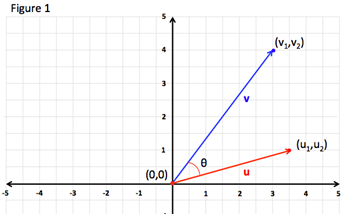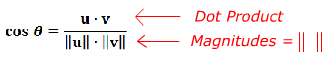# Angle between Two Vectors

The discussion on direction angles of vectors focused on finding the angle of a vector with respect to the positive x-axis. This discussion will focus on the angle between two vectors in standard position. A vector is said to be in standard position if its initial point is the origin (0, 0).

Figure 1 shows two vectors in standard position.The angle between two vectors in standard position can be calculated as follows:

ANGLE BETWEEN TWO VECTORS:

If θ is the angle between two non-zero vectors in standard position u and v:Where $0\le \theta \le 2\pi$ and $\parallel \text{v}\parallel =\sqrt{{v}_{1}{}^{2}+{v}_{2}{}^{2}}$

Let's look at some examples.

To work these examples requires the use of various vector rules. If you are not familiar with a rule go to the associated topic for a review.

Example 1: Find the angle θ between $u=〈6,3〉$ and $v=〈5,13〉$.
 Step 1: Find the dot product of the vectors. Remember the result will be a scalar. $u·v={u}_{1}{v}_{1}+{u}_{2}{v}_{2}$ $u·v$ $\left(6·5\right)+\left(3·13\right)$ $30+39={69}$ Step 2: Find the magnitudes of each vector. $\parallel v\parallel =\sqrt{{v}_{1}{}^{2}+{v}_{2}{}^{2}}$ ||u|| = $\sqrt{{u}_{1}{}^{2}+{u}_{2}{}^{2}}$ ||u|| = $\sqrt{{6}^{2}+{3}^{2}}$ ||u|| = $\sqrt{45}={3}\sqrt{5}$ ______________________________ ||v|| = $\sqrt{{v}_{1}{}^{2}+{v}_{2}{}^{2}}$ ||v|| = $\sqrt{{5}^{2}+{13}^{2}}$ ||v|| = $\sqrt{194}$ Step 3: Substitute and solve for θ. $\theta ={\mathrm{cos}}^{-1}\frac{23}{\sqrt{970}}$ $\theta \approx {42}{°}$
Example 2: Find the angle θ between $u=〈3,-6〉$ and $v=〈8,4〉$.
 Step 1: Find the dot product of the vectors. Remember the result will be a scalar. $u·v={u}_{1}{v}_{1}+{u}_{2}{v}_{2}$ $u·v$ $\left(3·8\right)+\left(-6·4\right)$ $24-24={0}$ Step 2: Find the magnitudes of each vector. $\parallel v\parallel =\sqrt{{v}_{1}{}^{2}+{v}_{2}{}^{2}}$ ||u|| = $\sqrt{{u}_{1}{}^{2}+{u}_{2}{}^{2}}$ ||u|| = $\sqrt{{3}^{2}+{\left(-6\right)}^{2}}$ ||u|| = $\sqrt{45}={3}\sqrt{5}$ ______________________________ ||v|| = $\sqrt{{v}_{1}{}^{2}+{v}_{2}{}^{2}}$ ||v|| = $\sqrt{{8}^{2}+{4}^{2}}$ ||v|| = $\sqrt{80}={2}\sqrt{20}$ Step 3: Substitute and solve for θ. As soon as you determine that the dot product is 0 you do not need to calculate the magnitudes. They are completed here for your benefit. Note that when two vectors in standard position have a dot product of 0 the angle between them is 90°. $\theta ={\mathrm{cos}}^{-1}0$ $\theta ={90}{°}$

 Related Links: Math algebra Decomposing a Vector into Components The First Derivative Rule Pre Calculus

To link to this Angle between Two Vectors page, copy the following code to your site: